Homework Help Question & Answers

# Consider an enzyme with the following properties: – Km = 0.0050 M At what substrate conc....

Consider an enzyme with the following properties: – Km = 0.0050 M

At what substrate conc. would the enzyme operate at one-fourth of its maximum rate?

At a given substrate conc. (e.g., ½Km) what would the rate be as a fraction of Vmax?

#### Homework Answers

Answer #1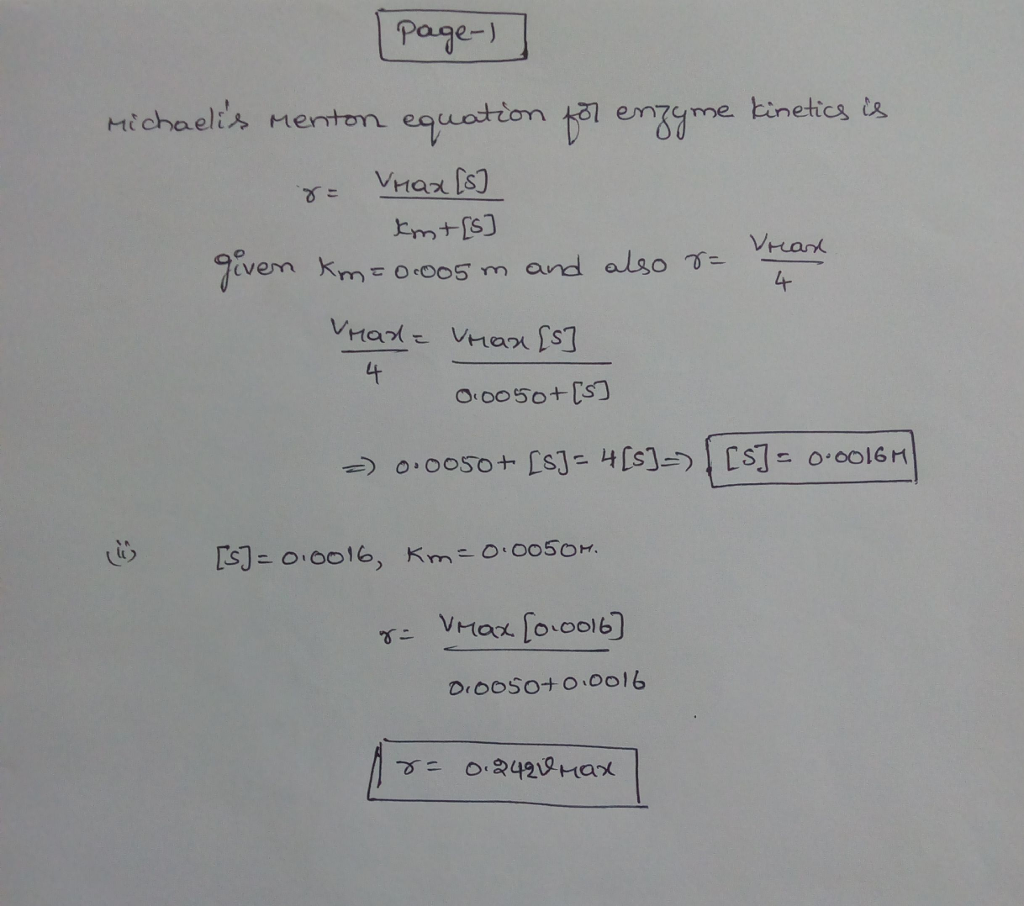Know the answer?
Your Answer:

#### Post as a guest

Your Name:

What's your source?

#### Earn Coin

Coins can be redeemed for fabulous gifts.

Not the answer you're looking for? Ask your own homework help question. Our experts will answer your question WITHIN MINUTES for Free.
Similar Homework Help Questions
• ### The relation between Reaction Velocity and Substrate Concentration: Michaelis-Menten Equation a) At what substrate concentration would...

The relation between Reaction Velocity and Substrate Concentration: Michaelis-Menten Equation a) At what substrate concentration would an enzyme with a kcat of 30.0 s-1 and a Km of 0.0050 M operate at one-quarter of its maximum rate? b) Determine the fraction of Vmax that would be obtained at the following substrate concentrations: [S]=Km/2, [S]=2Km, [S]=10Km

• ### 5. The Km of an enzyme of an enzyme-catalyzed reaction is 6.5 uM. What substrate concentration...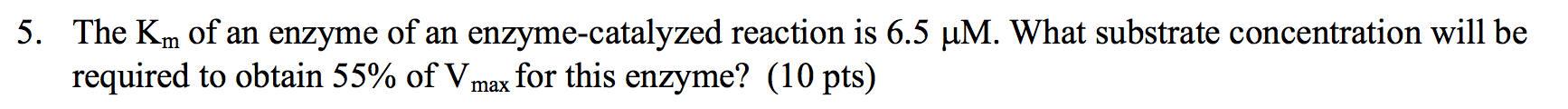5. The Km of an enzyme of an enzyme-catalyzed reaction is 6.5 uM. What substrate concentration will be required to obtain 55% of Vmax for this enzyme? (10 pts)

• ### Estimation of Vmax and K by Inspection Although graphical methods are available for accurate determination of...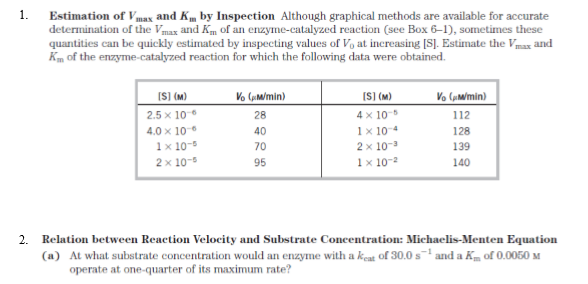Estimation of Vmax and K by Inspection Although graphical methods are available for accurate determination of the Vmax and K of an enzyme-catalyzed reaction (see Box 6-1), sometimes these quantities can be quickly estimated by inspecting values of Vat increasing (Sl. Estimate the Vmax and Km of the enzyme-catalyzed reaction for which the following data were obtained. V (pm/min) V (m/min) 112 [S] (M) 2.5 x 10 4.0 x 100 1 x 10-5 2 x 10-5 [S(M) 4 x 10-5...

• ### For an enzyme that follows the Michaelis-Menten kinetic, what substrate concentrations (relative to Km) are needed...

For an enzyme that follows the Michaelis-Menten kinetic, what substrate concentrations (relative to Km) are needed for the speed of the reaction to be 0.12 there vmax 0.25 there vmax 0.5 there vmax 0.9 there vmax.

• ### What is substrate concentration, expressed as a multiple of Km, when an enzyme reaction is observed...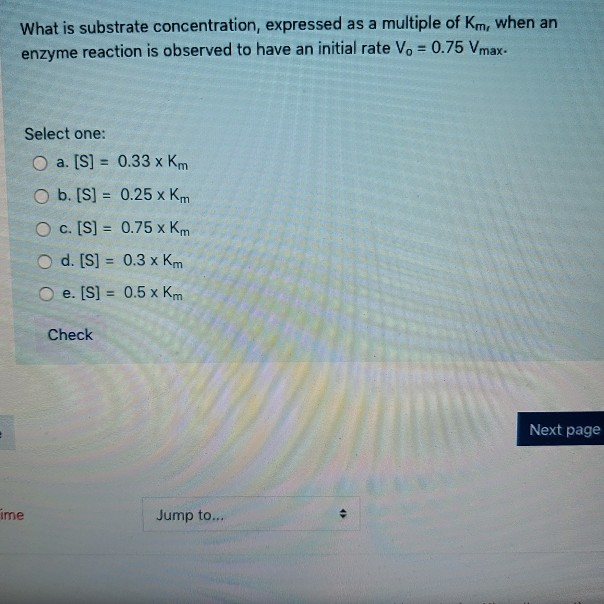What is substrate concentration, expressed as a multiple of Km, when an enzyme reaction is observed to have an initial rate Vo = 0.75 Vmax. Select one: O a. [S] = 0.33 x km O b. [S] = 0.25 km O c. [S] = 0.75 x Km O d. [S] = 0.3 x km O e. [S] = 0.5 x km Check Next page ime Jump to...

• ### An enzyme catalyzes the reaction M↽−−⇀N . An enzyme catalyzes the reaction M = N. The...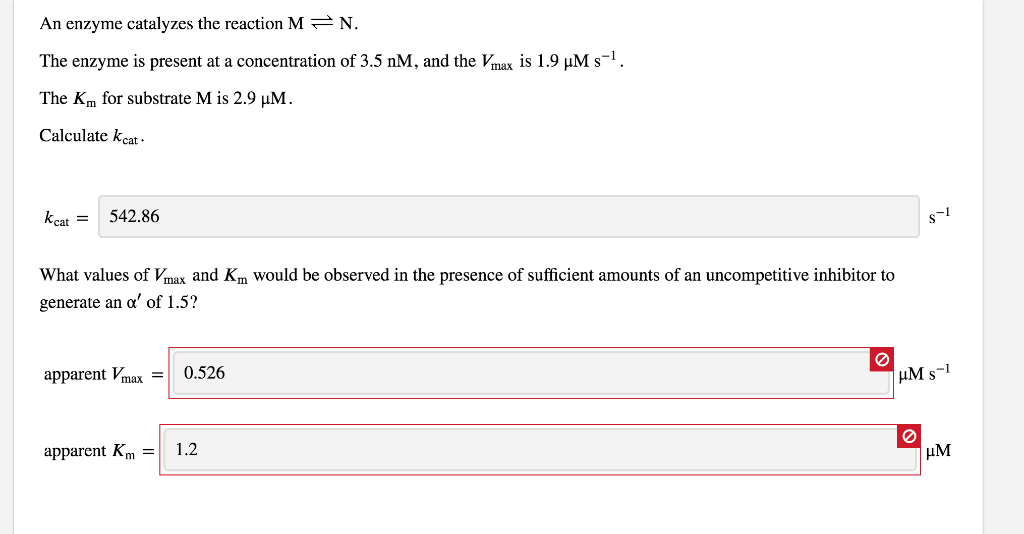An enzyme catalyzes the reaction M↽−−⇀N . An enzyme catalyzes the reaction M = N. The enzyme is present at a concentration of 3.5 nM, and the Vmax is 1.9 uM -. The Km for substrate M is 2.9 uM. Calculate kcat kcat = 542.86 What values of Vmax and Km would be observed in the presence of sufficient amounts of an uncompetitive inhibitor to generate an a' of 1.5? apparent Vmax = 0.526 UM s-1 apparent Km = 1.2

• ### An enzyme catalyzes the reaction M N. The enzyme is present at a concentration of 3.5...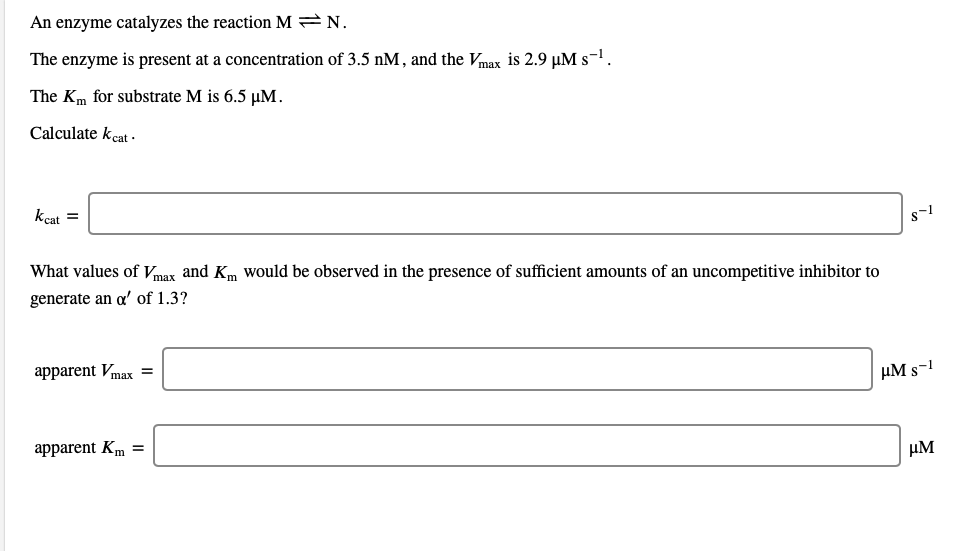An enzyme catalyzes the reaction M N. The enzyme is present at a concentration of 3.5 nM, and the Vmax is 2.9 PM s-. The Km for substrate M is 6.5 MM. Calculate kcat kcat = 1 What values of Vmax and Km would be observed in the presence of sufficient amounts of an uncompetitive inhibitor to generate an a' of 1.3? apparent Vmax = UM S-1 apparent Km = UM

• ### An enzyme has a maximum velocity of 48 nmoles substrate converted per sec. In the presence of 4 M inhibitor, Vmax is 36...

An enzyme has a maximum velocity of 48 nmoles substrate converted per sec. In the presence of 4 M inhibitor, Vmax is 36 nmoles substrate converted per sec with no change in Km. Determine the Ki of the inhibitor. Identify the type of inhibitor with explanation.

• ### 7. a) In an enzyme catalyzed reaction which follows the Michaelis-Menten kinetics. The substrate ...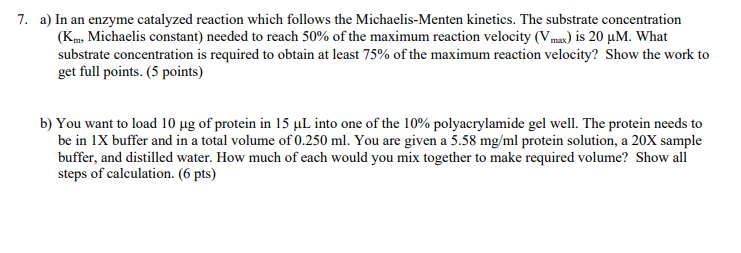7. a) In an enzyme catalyzed reaction which follows the Michaelis-Menten kinetics. The substrate concentration (Km, Michaelis constant) needed to reach 50% of the maximum reaction velocity (Vmax) is 20 μΜ. What substrate concentration is required to obtain at least 75% of the maximum reaction velocity? Show the work to get full points. (5 points) b) You want to load 10 μg of protein in 15 μL into one of the 10% polyacrylamide gel well. The protein needs to be...

• ### If the enzyme concentration is 3.8 X 10-8, what concentration of substrate would generate a velocity...

If the enzyme concentration is 3.8 X 10-8, what concentration of substrate would generate a velocity equal to 0.25 Vmax? Km is not needed.

Free Homework App

Scan Your Homework
to Get Instant Free Answers
Need Online Homework Help?

Get Answers For Free
Most questions answered within 3 hours.